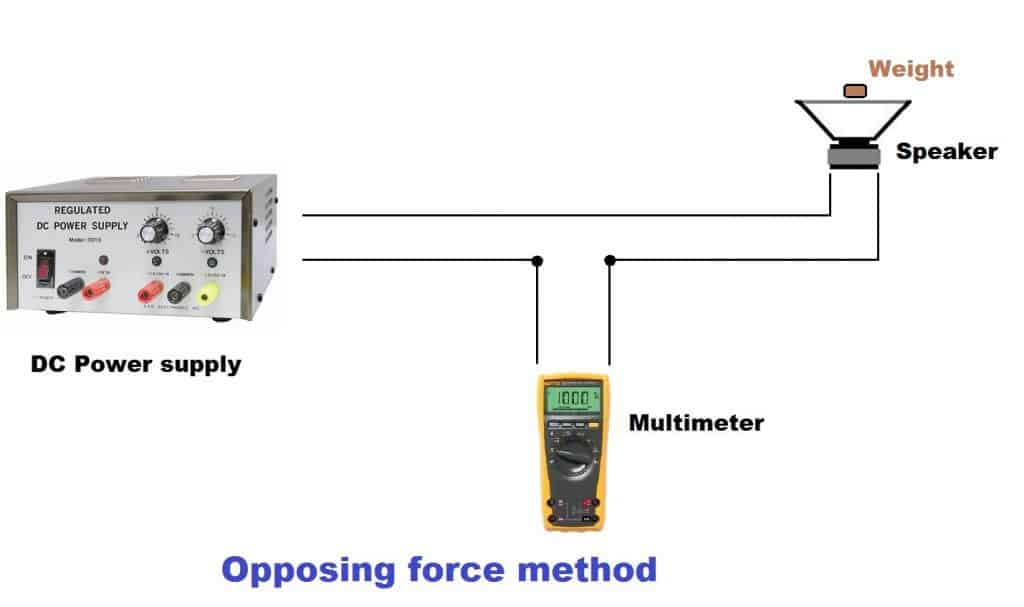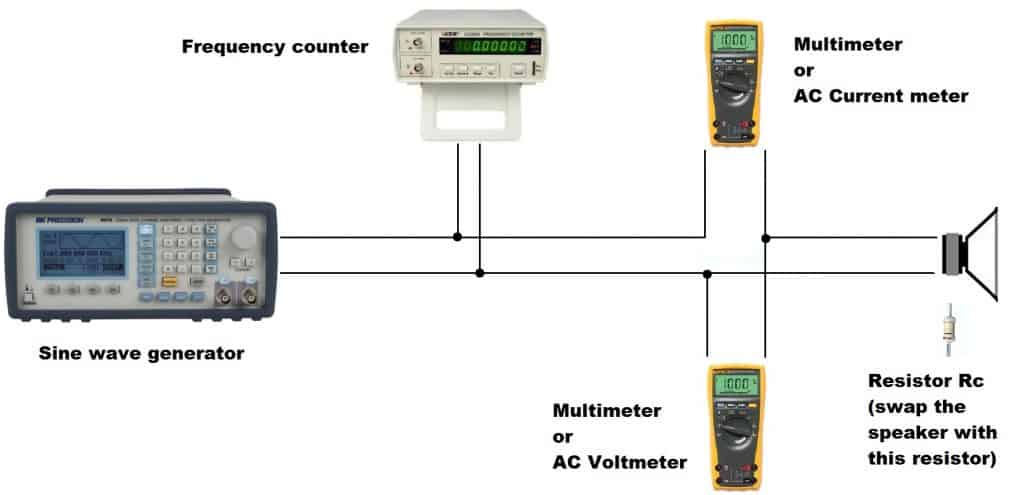## How to calculate Thiele Small parameters Episode 2

This is the second part of “How to measure Thiele Small parameters” article. Make sure that you read the first article, by clicking here. Since there is no need for any other introductions, I’ll go straight to the next TS parameter.

### How to measure speaker BL (motor strength) ?

Equipment needed (Amazon affiliate links) :

BL is actually B * L, which is the produce between the magnetic field density and the length of the wire (from the voice coil) in the magnetic field. There are several ways to measure the BL of a speaker, but the most popular method is the opposing force method.Before you start making any adjustments to the equipment, you need to place the speaker horizontally on a flat, stable surface. Next, place an object on the cone, which has a known mass (Ma). Most noteworthy, the mass needs to be known precisely (down to 0.1 grams). The object needs to be sufficiently heavy to depress the the cone at least 0.25 ” (0.65 cm). Then, you will have to apply increasing DC voltage to the speaker, until the cone reaches its starting position. Basically, you are applying voltage to the speaker, to fight the weight of the object on the cone. If you are increasing the voltage and the speaker goes further down, you need to reverse the polarity. When the speaker reached the original resting position (with the object on the cone), note the current (I) reading on the multi-meter.

#### Motor strength is then calculated by the formula :

BL = (9.8 * Ma) / I

Units of measurement: BL in tesla meters, Ma in kilograms, and I in amperes.

The hard part of this method is to accurately determine the rest position of the speaker before the adding the weight. Furthermore, another challenge is to stop at the exact same location after applying current. A cheap and easy way to do this, is to get a small object (a needle for example). Suspend it in the air on a string so it’s just touching the speaker cone, on the sharp side. After that, you place the weight on the cone. It will create a gap between the needle and the speaker cone. Now you have to apply voltage to the speaker, until the cone just barely touches the needle again. You can get creative with the logistics of this method.

### How to measure speaker Q (Qms and Qes) ?

There are a few ways to measure driver’s Q. One method is suggested by Mr. Thiele, which finds the -3 db points on either side of the slope of the resonance peak of the speaker. Another method is to calculate Qms and Qes, in the case that BL and Cms are given, or measured beforehand. First method, while largely accepted, is susceptible to measurement errors, because of nonlinear driver surrounds. In conclusion, the second method will yield more accurate results. Of course, there are other methods, besides these, which are more accurate, but involve expensive equipment.

#### Method 1

Equipment needed (Amazon affiliate links) :#### By using the setup described above, follow these steps :

1. Measure the DC resistance of the speaker (Re). Set your multi-meter to ohms, and take a reading at the speaker terminals.
2. Choose a resistor (Rc) with has a close resistance value to Re. If Re is 6.7 ohms, an 8 ohm resistor will be close enough.
3. Swap the speaker with the resistor Rc. Set the sine wave generator to the resonance frequency (fs). Set the voltage to a small value, around 100 mV. If this does not provide good results with your equipment, you can go higher to 200 – 700 mV, which is still small signal range. Take note of this voltage. The value is not important, but you must check upon it, at every step, as it must remain the same.
4. After you set up the standard voltage, and your generator is emitting the resonant frequency, take a reading at the current meter and label it Ic.
5. Calculate : Ie = (Ic * Rc) / Re
6. Take the resistor Rc off and put the speaker back. Attach the speaker to an open frame or hold it in your hand. Set the generator for minimum current, which will be fs. This minimum current at fs is labeled I0.
7. Calculate : r0 = Ie / I0
8. Calculate : Ir = (Ie * I0)1/2
9. Find f1 and f2, which are positioned below and above fs, whose current are equal to Ir. It is important to check if your measurement is correct, by calculating fs = (f1 * f2)1/2 . If the measured value is less or within 1 Hz of the calculated value, then it can be considered an accurate measurement.
10. Qms = (fs * r01/2) / (f2 – f1)
11. Qes = Qms / (r0 – 1)
12. Total Q is calculated by adding Qes to Qms, like adding resistances in parallel : Qts = (Qes * Qms) / (Qes + Qms)

#### Method 2

This method is based on a calculation, and needs Cms and BL to be given, or measured beforehand.

First, take a look back at part 1 of this article, and read again the impedance section. Now calculate Re, and the resistance of the speaker at resonance (fs), which we will label R. After that, calculate Res:

Res = R – R(ohms)

Then you can calculate Qms:

Qms = Res / (BL2 * Cms * 6.283 * fs)

And Qes :

Qes = Re / (BL2 * Cms * 6.283 * fs)

Besides these 2 methods, there are other ones which require expensive equipment. To name a few : Klippel, Dumax, Audio Precision APx series. These are professional equipment that measure some Thiele Small parameters with a click of a button.

### How to measure speaker Vas ?

Out of all the thiele small parameters, Vas is one of the most difficult to measure. Furthermore, its value can change in a significant way, just by having a different air temperature or humidity. Out of all the methods to measure Vas, I am going to list 2 of them, which are the easiest.

#### Method 1

Make a sealed box. If you woofer is 8″ or 10″, make it 1 ft3. If you woofer is 12″ or 15″, then make it 2 ft3. This volume will be labeled Vt. Make sure to leave the box empty, and not place any damping material inside. Also, make sure you attach the speaker with the magnet sticking outside of the box, that way you don’t have to add the volume displaced by the magnet assembly, to the overall volume.

### How to design loudspeakers - video courses

After that, calculate the fs and Qes of the driver in free-air. These 2 are explained in the first part of the article and just above. Now calculate the same Thiele Small parameters, but with the speaker attached to the box. These parameters are labeled fc (resonant frequency of the box) and Qect.

Calculate Vas using this formula :

Vas = Vt * {[(fc * Qect) / (fs * Qes)] -1}

#### Method 2

This method needs Cms (driver compliance) and Sd (aria of the driver) values. Then, use this formula :

Vas = 142000 * Sd2 * Cms

This second method is more reliable because it is independent of driver leakage losses. However, because it is dependent of Cms, it means that it is subject to suspension non-linearities, which could translate into measurement errors. However, using very low voltage on your measuring equipment, will reduce this drawback.

### How to measure speaker inductance (Le) ?

Using the setup to map out the impedance, which is described in part 1 of this article, set your sine wave generator to 10 kHz and note the impedance magnitude (m).

Calculate voice coil inductance using this formula :

Le = 1.592 * 10-5 * (m2 – Re2)1/2

### Conclusion

The Thiele Small parameters are quite difficult to comprehend. As a result, only after some time you will get the hang of it, and how they interact with each other. Usually, when one is modified, a few others change their value as a result of that action. Learning how to measure them, and working with real numbers, will give you a more practical approach in understanding how they work by themselves, and how they influence one another. The Thiele Small parameters are the core of a good speaker design and/or a good enclosure design.

#### References

1. Loudspeaker Design Cookbook 7th Edition by Vance Dickason (Audio Amateur Pubns, 2005). (Amazon affiliate link)